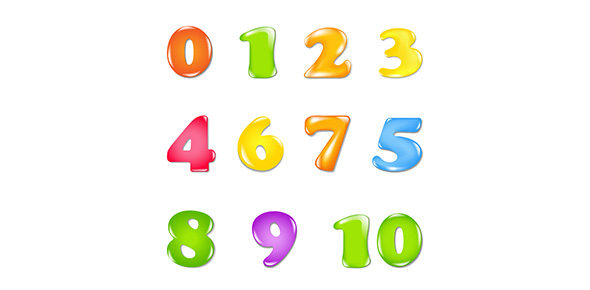10 Questions | Total Attempts: 1121SettingsThis quiz will test your foundation skills in addition. If you pass it without the use of a calculator, then you're off to a good start.

• 1.
Which of these symbols is used to represent addition?
• A.

+

• B.

-

• C.

X

• D.

÷

• 2.
What are some other words for addition? (Select all that apply)
• A.

Plus

• B.

Decrease

• C.

Total

• D.

Dividend

• E.

Increase

• 3.
Addition means to put one group of items together with other items and then counting to see how many items there are all together. True of False?
• A.

True

• B.

False

• 4.
3 + 9 =
• 5.
What is the first step to solving an addition question?
• A.

• B.

Get a calculator

• C.

Rewrite the question, lining up the digits from the right.

• 6.
Without a calculator, add the folowing numbers together:56 + 39 =
• 7.
Without a calculator, add the folowing numbers together:725 + 6793 =
• 8.
Without a calculator, add the folowing numbers together:80536 + 4596 =
• 9.
Without a calculator, add the folowing numbers together:109284 + 35673 =
• 10.
Without a calculator, add the folowing numbers together:9144957 + 85123 =
Related TopicsBack to top